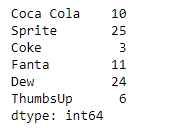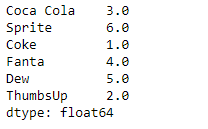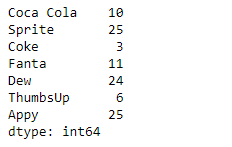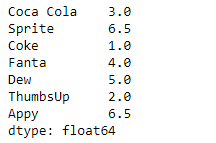Open In App

# Python | Pandas Series.rank()

Pandas series is a One-dimensional ndarray with axis labels. The labels need not be unique but must be a hashable type. The object supports both integer- and label-based indexing and provides a host of methods for performing operations involving the index.

Pandas` Series.rank()` function compute numerical data ranks (1 through n) along axis. Equal values are assigned a rank that is the average of the ranks of those values.

Syntax: Series.rank(axis=0, method=’average’, numeric_only=None, na_option=’keep’, ascending=True, pct=False)

Parameter :
axis : index to direct ranking
method : {‘average’, ‘min’, ‘max’, ‘first’, ‘dense’}
numeric_only : Include only float, int, boolean data. Valid only for DataFrame or Panel objects
na_option : {‘keep’, ‘top’, ‘bottom’}
ascending : False for ranks by high (1) to low (N)
pct : Computes percentage rank of data

Returns : ranks : same type as caller

Example #1: Use `Series.rank()` function to rank the underlying data of the given Series object.

 `# importing pandas as pd``import` `pandas as pd`` ` `# Creating the Series``sr ``=` `pd.Series([``10``, ``25``, ``3``, ``11``, ``24``, ``6``])`` ` `# Create the Index``index_ ``=` `[``'Coca Cola'``, ``'Sprite'``, ``'Coke'``, ``'Fanta'``, ``'Dew'``, ``'ThumbsUp'``]`` ` `# set the index``sr.index ``=` `index_`` ` `# Print the series``print``(sr)`

Output :Now we will use `Series.rank()` function to return the rank of the underlying data of the given Series object.

 `# assign rank``result ``=` `sr.rank()`` ` `# Print the result``print``(result)`

Output :As we can see in the output, the `Series.rank()` function has assigned rank to each element of the given Series object.

Example #2: Use `Series.rank()` function to rank the underlying data of the given Series object. The given data also contains some equal values.

 `# importing pandas as pd``import` `pandas as pd`` ` `# Creating the Series``sr ``=` `pd.Series([``10``, ``25``, ``3``, ``11``, ``24``, ``6``, ``25``])`` ` `# Create the Index``index_ ``=` `[``'Coca Cola'``, ``'Sprite'``, ``'Coke'``, ``'Fanta'``, ``'Dew'``, ``'ThumbsUp'``, ``'Appy'``]`` ` `# set the index``sr.index ``=` `index_`` ` `# Print the series``print``(sr)`

Output :Now we will use `Series.rank()` function to return the rank of the underlying data of the given Series object.

 `# assign rank``result ``=` `sr.rank()`` ` `# Print the result``print``(result)`

Output :As we can see in the output, the `Series.rank()` function has assigned rank to each element of the given Series object. Notice equal values has been assigned a rank which is the average of their ranks.